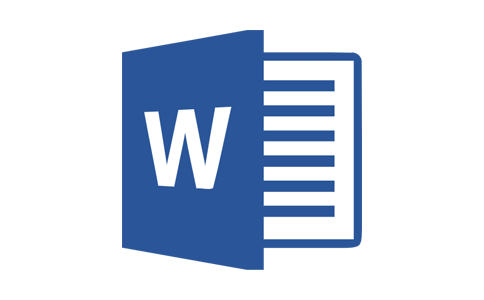# 三年级数学加减法口算练习题2

2021-05-22 17:48:23来源：未知作者： admin阅读量：27+46=
90+47=
18+15=
92+65=
18+53=
29+32=
14+56=
72+76=
71+68=
39+79=
64+60=
38+69=
83+62=
12+98=
17+84=
175+157=
645+239=
808+213=
256+775=
607+914=
352+708=
766+792=
970+602=
343+801=
916+861=
806+487=
691+400=
106+537=
353+939=
328+114=
810－125=
648－585=
398－148=
716－117=
704－124=
449－312=
713－288=
202－155=
954－286=
802－668=
860－162=
287－167=
859－371=
941－343=
472－425=
900－168=
300－186=
900－554=
800－200=
700－666=
300－122=
900－353=
500－162=
900－705=
400－133=
200－158=
300－257=
400－157=
700－205=
300－288=